# NMAT Mock Test - 6

## 120 Questions MCQ Test NMAT Mock Test Series | NMAT Mock Test - 6

Description
Attempt NMAT Mock Test - 6 | 120 questions in 120 minutes | Mock test for CAT preparation | Free important questions MCQ to study NMAT Mock Test Series for CAT Exam | Download free PDF with solutions
QUESTION: 1

### Answer the following question based on the information given below. The graph below gives details of total exports as well as exports of herbal products for a company. The abscissa represents the total exports for a given year in Rs. Lakhs, while the ordinate represents the exports of herbal products for that same year in Rs. Lakhs.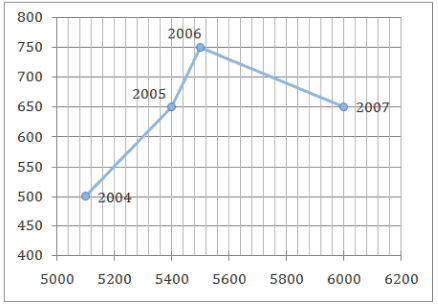Q.For 2006, herbal products formed what percentage of the total exports?

Solution:

The abscissa and ordinate stand for the X-axis and Y-axis respectively.
Thus, figures on the X-axis stand for the total exports for a given year while those on the Y-axis stand for the export of herbal products in that year.
Thus, in 2006, the total exports were Rs. 5,500 lakhs, whereas the exports of herbal products were Rs. 750 lakhs.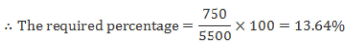Hence, option 2.

QUESTION: 2

### The graph below gives details of total exports as well as exports of herbal products for a company. The abscissa represents the total exports for a given year in Rs. Lakhs, while the ordinate represents the exports of herbal products for that same year in Rs. Lakhs.Q.The fall in herbal exports in 2007 from that in 2006 was nearly:

Solution:

In 2006, the herbal exports were Rs. 750 lakhs, whereas in 2007, they were Rs. 650 lakhs.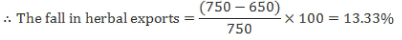Hence, option 3.

QUESTION: 3

### The graph below gives details of total exports as well as exports of herbal products for a company. The abscissa represents the total exports for a given year in Rs. Lakhs, while the ordinate represents the exports of herbal products for that same year in Rs. Lakhs.Q.Over the given period, the herbal exports rose by nearly:

Solution:

In 2004, the herbal exports were Rs. 500 lakhs while at the end of the period, i.e. in 2007, they were Rs. 650 lakhs.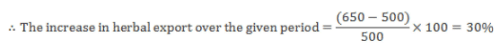Hence, option 2.

QUESTION: 4

The graph below gives details of total exports as well as exports of herbal products for a company.
The abscissa represents the total exports for a given year in Rs. Lakhs, while the ordinate represents the exports of herbal products for that same year in Rs. Lakhs.Q.The average percentage increase in total exports was:

Solution:

The total export in 2004 were Rs. 5100 lakhs, while in 2007, they were Rs. 6000 lakhs.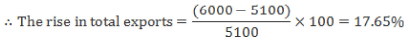This rise is over a period of 3 years.
The average percentage increase in export = 17.65/3 = 5.88% Hence, option 4.

QUESTION: 5

The ratio of cost price and marked price of an article is 2 : 3. The ratio of percentage profit and percentage discount is 3 : 2. What is the discount percentage?

Solution:

Cost price : Marked price = 2 : 3 and Profit % : Discount% = 3 : 2

Let cost price, (C.P.) = 2x; marked price, (M.P.) = 3x; Profit % = 3y and Discount % = 2y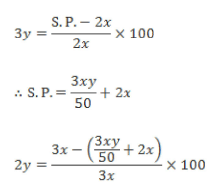Solving this, we get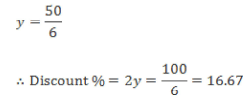Hence, option 1.

QUESTION: 6

Jai and Veeru are two masons who are given the job of building a compound wall around a plot owned by Mr. Thakur. Jai working alone takes 8 hours more than the time that both Jai and Veeru would take working together. Veeru working alone takes 12.5 hours more than the time that both would take working together. How many hours would Jai alone take to build the wall?

Solution:

Let the time taken to build the compound when both Jai and Veeru are working together be x.

Time taken by Jai to complete the job alone is x + 8 and the time taken by Veeru to complete the job alone is x + 12.5 Thus we get the following equation: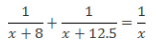2x2 + 20.5x — x2 + 20.5x +100

x2 = 100

x = 10
Thus Jai will take 18 hours to complete the job alone.
Hence, option 2.

QUESTION: 7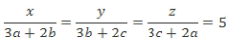where a, b and c are in continued proportion and b, c and a are in continued proportion.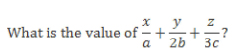Solution:

a, b and c are in continued proportion.

b2 = ac ...(i)

b, c and a are in continued proportion. c2 = ab •••(ii)

Multiplying (i) and (ii), we get,

a2bc = c2b2

a2 = be ...(iii)

All three will be satisfied only when a = b = c = k (say)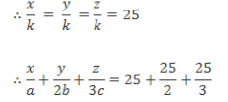= 45.83
Hence, option 1.

QUESTION: 8

A number which reads the same when read forward and backward is called a palindrome.

Q.How many four-digit numbers are palindromes?

Solution:

A 4-digit palindrome will be of the form abba where a > 0 and b can take integer values from 0 to 9.

a can take 9 values and b can take 10 values.

The total number of 4-digit palindromes is 9 x 10 = 90 Hence, option 2.

QUESTION: 9

The sum of a two-digit number and the number formed by interchanging the two digits is 45 more than twice the original number. If the sum of the digits of the number is 9, what is the original number?

Solution:

Let the original two digit number be xy. 10x + y + l0y + x = 40 + 2(10x + y)

11x + 1 ly = 45 + 20x + 2y

9y - 9x = 45

y-x = 5 ... (I)

Also, y + x = 9 ... (II)

Solving (I) and (II), y = 7 and x = 2 Hence, the original number is 27.
Hence, option 5.

QUESTION: 10

Group Question

Answer the following question based on the information given below.

A company with pan-India presence has segregated its operations into four regions - North, East, West and South. For tracking purposes, each region is further divided into two sub-regions i.e. 1 and 2. e.g. North is divided into North 1 and North 2, and so on. The bar-graph below shows the percentage distribution of male and female employees of the company in these eight sub-regions.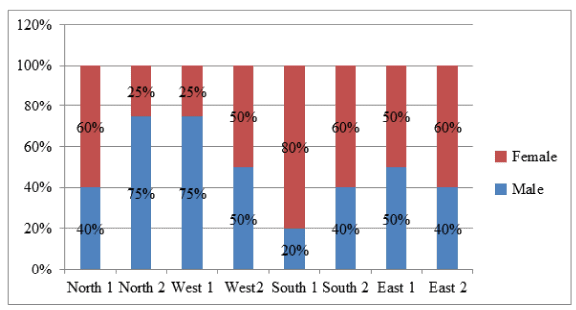The percentage of male employees in the North, West, South, and East regions is 60%, 55%, 30% and 45% respectively.

Q.The ratio of total employees in North 1 to North 2 is

Solution:

Number of males in North 2 = 0.756b And, number of males in North = 0.6(a + b)

0.4a + 0.756 = 0.6(a + 6)

0.4a + 0.756 = 0.6a + 0.66

0.2a = 0.156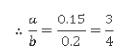Hence, option 1.

QUESTION: 11

A company with pan-India presence has segregated its operations into four regions - North, East, West and South. For tracking purposes, each region is further divided into two sub-regions i.e. 1 and 2. e.g. North is divided into North 1 and North 2, and so on. The bar-graph below shows the percentage distribution of male and female employees of the company in these eight sub-regions.Q.If there are 500 employees in West, how many male employees does West 2 have?

Solution:

Number of male employees in West = 55% of 500 = 275

Let there be a employees in West 1 and (500 - a) employees in West 2.

Number of male employees in West 1 = 0.75a

And, number of male employees in West 2 = 0.5(500 - a)

0.75a+ 0 .5(500 - a) = 275

0.75a + 250 - 0.5a = 275

0.25a = 25

a = 100
Number of male employees in West 2 = 0.5(500 - 100) = 200

Hence, option 1.

QUESTION: 12

A company with pan-India presence has segregated its operations into four regions - North, East, West and South. For tracking purposes, each region is further divided into two sub-regions i.e. 1 and 2. e.g. North is divided into North 1 and North 2, and so on. The bar-graph below shows the percentage distribution of male and female employees of the company in these eight sub-regions.Q.If there are 60 male employees in South 2, what is the total number of employees in South?

Solution:

Let the total employees in South be a.
Since there are 60 male employees in South 2,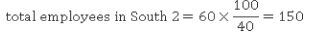Total employees in South 1 = a - 150. Total male employees in South 1 = 0.2(a - 150) = 0.2a - 30

Also, total male employees in South = 30% of a = 0.3a

0.2a - 30 + 60 = 0.3a

0.1a = 30

a -300

Hence, option 4

QUESTION: 13

A company with pan-India presence has segregated its operations into four regions - North, East, West and South. For tracking purposes, each region is further divided into two sub-regions i.e. 1 and 2. e.g. North is divided into Northl 1 and North 2, and so on. The bar-graph below shows the percentage distribution of male and female employees of the company in these eight sub-regions.Q.The ratio of female employees in East 1 to East 2 is

Solution:

Let there be 100 employees in East Number of female employees in East = 55% of 100 = 55

Now, let a employees from East be in East 1 and the remaining (100 - a) be in East 2.

Number of female employees in East 1 = 50% of a = 0.5a

And, number of female employees in East 2 = 60% of (100 - a) = 0.6(100 - a)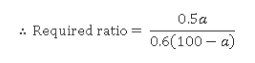Now, 0.5a + 0.6(100 - a) = 55 0.5a + 60 - 0.6a = 55

0.1a = 5

a = 50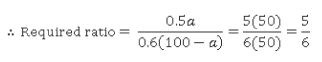Hence, option 2.

QUESTION: 14

Group Question

Study the table given below and answer the questions that follow:

The table below shows the cours-wise and section-wise breakup of students in a university.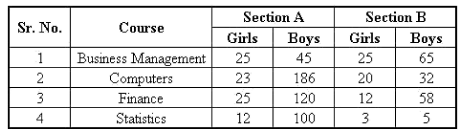Q.If all the boys and 80% of all the girls are successful in the courses taken by them, what is the combined passing percentage of the university?

Solution:

Total girls in the university = 25 + 23 + 25 + 12 + 25 + 20 + 1 2 + 3 = 145.
Total boys in the university = 45 + 186 + 120 + 100 + 65 + 32 + 58 + 5 = 611.
Total students = 145 + 611 = 756

Number of students who passed = 611 + 0.8(145) = 727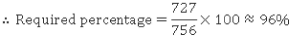Hence, option 5.

QUESTION: 15

The table below shows the cours-wise and section-wise breakup of students in a university.Q.In which course is the number of girls as a percentage of the total students in that course the highest?

Solution:

Business Management has 50 girls and 110 boys i.e. 50 girls in 160 students Computers has 43 girls and 218 boys i.e. 43 girls in 261 students Finance has 37 girls and 178 boys i.e. 37 girls in 215 students Statistics has 15 girls and 105 boys i.e. 15 girls in 120 students It can be directly observed that the number of girls as a percentage of total number of students in that course is the highest in Business Management (31.25%)
Hence, option 1.

QUESTION: 16

The table below shows the cours-wise and section-wise breakup of students in a university.Q.By what percentage is the number of students pursuing a course in Computers more than the number of students pursuing Finance?

Solution:

The number of students pursuing Computers = 23 + 186 + 20 + 32 = 261 The number of students pursuing Finance = 25 + 120 + 12 + 58 = 215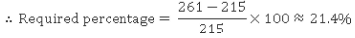Hence, option 5.

QUESTION: 17

The table below shows the cours-wise and section-wise breakup of students in a university.Q.The number of successful students in the Computers course is thrice the number of successful girl students in the Business Management course. If the number of successful girl students in the Business Management course is 46% of all the girls in this course, what is the percentage of successful students in the Computers course?

Solution:

The Business Management course has 50 girl students Number of successful girl students in the Business Management course = 46% of 50 = 23

Number of successful students in the Computers course = 3 x 23 = 69 The Computers course has 261 students.
Required percentage = 69/261 x 100 = 26.43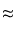26%

Hence, option 4.

QUESTION: 18

D, E, F are the mid points of the sides BC, CA and AB respectively of a triangle ABC. What is the ratio of the circumradii of triangles DEF and ABC respectively?

Solution: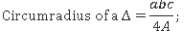where a, b, c are the sides of the triangle and A is the area of the triangle.
D, E, F are the mid points of the sides BC, CA and AB respectively of a triangle ABC.
Hence, from the mid-point theorem, the sides of ΔDEF are half the sides of Δ ABC and A( ΔDEF) is one-fourth the Δ(AABC)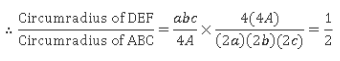Hence, option 2.

QUESTION: 19

In a plane there are two lines, a and b. a has 4 points on it while b has 5 points on it. Beside this there are six other points in this plane, no three of which are collinear. Also, apart from the points that lie on lines a and b, no three points are collinear. What is the maximum number of quadrilaterals that can be drawn using these points?

Solution:

In all there are 15 points in the plane. Number of ways in which four points can be selected from these 15 points is 15C4 = 1365.
But to form a quadrilateral, not more than 2 points can be on the same line.
So the cases when a selection of 4 out of 15 points, made in the above manner, will not result in a quadrilateral are:

Case (i):

When all the 4 points selected are on line a.
This selection can be made in 4C4 = 1 way.
Case (ii):

When all the 4 points selected lie on line b.
This selection can be made in 5C4 = 5 ways.
Case (iii):

When 3 points lie on line a, while the 4th point lies on either line b or is one of the 6 points in the plane.
This selection can be made in 4C3 x 5C3 + 4C3 x 6C1 = 44 ways.
Case (iv): When 3 points lie on line b, while the 4th point lies on either line a or is one of the 6 points in the plane.
This selection can be made in 5C3 x 4C3 + 5C3 x 6C1 = 100 ways.
So the total number o f quadrilaterals that can be formed is 1 3 6 5 - (1 + 5 + 44 + 100) =1215.

Hence, option 3.

QUESTION: 20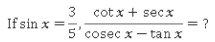Solution: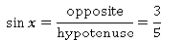If the side opposite to ∠x is 3p and the hypotenuse is 5p , the adjacent side has to be 4p .
Hence, using the standard definitions of the given trigonometric ratios,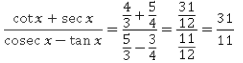Hence, option 4.

QUESTION: 21

Raman gives Suman a head start of 35 m in a 200 m race but still beats Suman by 45 m. In a 100 m race, both start simultaneously from the same point. By how many metres does Raman beat Suman in the 100 m race?

Solution:

In a 200 m race, Raman gives Suman a head start of 35 m but still beats him by 45 m.
So, Raman starts when Suman has covered 35 m and when Raman completes the race, Suman is yet to cover 45 m i.e. Suman has covered 155 m.
So, in the same time that Raman covers 200 m, Suman only covers 155 - 35 = 120 m.

So, Raman covers 100 m in the same time that Suman covers 60 m.
Therefore, when both start together in a 100 m race, Suman has only covered 60 m when Raman completes the race.
So, Raman beats Suman by 100 - 60 = 40 m.
Hence, option 1.

QUESTION: 22

Two friends, A and B, decide to play a game. Each friend has the same probability of winning a single game i.e. p. If the amount at stake is Rs. 22, and A is expected to win Rs. 12 if he starts first, what is the value of p ?

Solution:

Expected winning = probability of winning x amount at stake.
P (A winning) = p + (1 - p )( 1 - p )p + (1 - p )(1 - P )(1 -p)(1- p)p + •••

= p + (1 - p )2p + (1 - p)4p + ...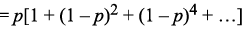The expression in the brackets is an infinite G.R with a = 1 and r = (1 - p )2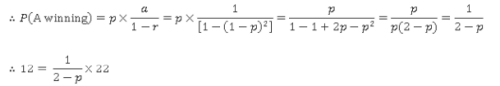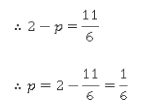Hence, option 5.

QUESTION: 23

A circular wire of area 4π sq.cm is bent into a square. The area of the square is what percentage of the area of the circle?

Solution:

Since the same wire is bent from a circle to a square, the circumference of the circle is equal to the perimeter of the square.
Let the radius of the circle measure r cm.

πr2 = 471

r = 2 cm

Perimeter of square = circumference of circle = 2πr = 4π

Side of square = 4π/4= π cm

Area of square = π2 sq.cm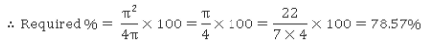Hence, option 3.

QUESTION: 24

The average of a, b and c is 113. What is the value of b, if c is as much more than the average as much as a is less than the average?

Solution: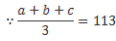a + b + c = 339 ...(i)

Now, c is as much more than the average as much as a is less than the average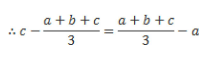c - 113 = 113 + a

a + c = 226 ...(ii)

Subtracting equation (ii) from equation (i), we get,
b = 113

Hence, option 3.
Alternatively,
The simplest way to solve this question is to understand that for an arithmetic progression, arithmetic mean of three numbers is equal to the middle term and the difference between the middle term and the first number is equal to the difference between the second term and the middle number.
b=113
Hence, option 3.

QUESTION: 25

A piece of work when done by Amar, Akbar and Anthony respectively, costs Rs. 540, Rs.324 and Rs. 320 respectively. The daily wages of Amar, Akbar and Anthony are Rs.36, Rs.18 and Rs.32 respectively. What will be the total cost when Amar, Akbar and Anthony are working together?

Solution:

Number of days required by Amar to complete the job alone = 540/36 = 15 days

Number of days required by Akbar = 324/18 = 18 days

Number of day srequired by Anthony = 320/32 = 10 days

The fraction of job that will be completed in a day when all three are working together is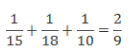4.5 days will be required to finish the job when all the three are working together.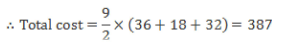Hence, option 3.

QUESTION: 26

What is the value of x in the equation below?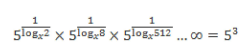Solution: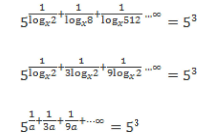Let logx2 = a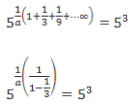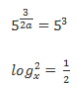From the above equation we get a = 1/2.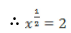x = 4

Hence, option 3.

QUESTION: 27

Five circles, each of radius 6 cm, are cut-out from a circular sheet of radius 14 cm. What is the ratio of the area of the remaining portion of the sheet to the area of the original sheet?

Solution:

Area of original sheet = π x 142 = 19671 sq.cm.
Area of the five circles = 5 x π x 62 = 180π sq.cm.
Area of the remaining portion of the sheet = 16π sq.cm.

Required ratio = 16π : 196π = 4 : 49 Hence, option 4.

QUESTION: 28

Each question is followed by two quantities, A and B. Answer each question using the following instructions:

Mark (1) if quantity A is greater than quantity B.
Mark (2) if quantity B is greater than quantity A.
Mark (3) if the two quantities are equal.
Mark (4) if it is impossible to determine a relationship.
Mark (5) if the greater quantity cannot be determined but the two quantities are definitely not equal.

In oodoo-dudu Island, coins are available only in two denominations - 4 Rupee and 5 Rupee. Rajesh had paid an amount of Rs. 150 to the shopkeeper.

A. The probability that the number of 4 rupee coins given by Rajesh is more than the number of 5 rupee coins.

B. The probability that the number of 5 rupee coins given by Rajesh is more than the number of 4 rupee coins.

Solution:

Let x be the number of 4 rupee coins that Rajesh paid andy be the number of 5 rupee coins that he paid.

4x + 5y - 150... (i)
Dividing (i) by 4 we get;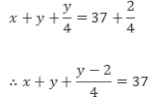Now, as x andy are number of coins, they should be integers.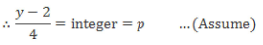y - 2 = 4p

y = 4p + 2 ...(ii)

Substituting (ii) in (i) we get,

4x + 5(4p + 2) = 150

4x = 150 - 10 - 20p

4x = 140 - 20p

x = 35 - 5p ...(iii)

For p > 7 , x will be negative and for p < 0, y will be negative.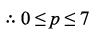Now for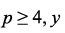will be greater than x and for p < 4, y will be less than x.

In no case will y be equal to x.
Probability that x > y = 4/8 = 0.5 and probability that x < y = 4/8 = 0.5.
Hence, both the quantities are equal. Hence, option 3.

Alternatively,

Let x be the number of 4 rupee coins that Rajesh paid and y be the number of 5 rupee coins that he paid.

4x + 5y = 150 ... (i)

5y = 150 - 4x

Since the LHS is a multiple of 5, the RHS has to be divisible by 5. Hence, 4x has to be a multiple of 5.
Hence, x can take 8 values i.e. 0, 5, 10, 15, 20, 25, 30 and 35.
The corresponding values ofy are 30, 36, 22, 18, 14, 10, 6 and 2.
Observe that for the first four values, x <y, and for the last four values, x >y

Probability that x > y = 4/8 = 0.5 and probability that x < y = 4/8 = 0.5.
Hence, both the quantities are equal.
Hence, option 3.

QUESTION: 29

Each question is followed by two statements, A and B. Answer each question using the following instructions:

Mark option (1) if the question can be answered by using statement A alone but not by using statement B alone.
Mark option (2) if the question can be answered by using statement B alone but not by using statement A alone.
Mark option (3) if the question can be answered by using either statement alone.
Mark option (4) if the question can be answered by using both the statements together but not by either of the statements alone.
Mark option (5) if the question cannot be answered on the basis of the two statements.

Q.Two quadratic equations 2x2 - 7x + k = 0 and x2 + x - 6 = 0 have a common root. What is the value of k?

A. k is a positive integer.
B. k + 39 is a natural number

Solution:

Solution: The roots of x2 + x - 6 = 0 are x = 2, -3

Let the common root of both equations be x = 2 & let the other root of 2x2 - 7x + k = 0 be a.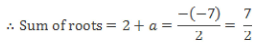a = 3/2

Also, product of roots =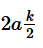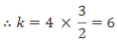Let the common root of both equations be x = -3 & let the other root of 2x2 - 7x + k = 0 be b.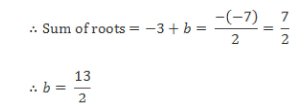Also, product of roots  = -3b = K/2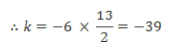Using statement A alone:

Since k is a positive integer, k = 6.
Thus, the question can be answered using statement A alone.

Using statement B alone:

k + 39 is a natural number

For k = 6 or - 39 , k + 39 = 45 or 0

0 is not a natural number.

k = 6.
Thus, the question can be answered using statement B alone.
Thus, the question can be answered using either statement alone.
Hence, option 3.

QUESTION: 30

Each question is followed by two statements, A and B. Answer each question using the following instructions:

Mark option (1) if the question can be answered by using statement A alone but not by using statement B alone.
Mark option (2) if the question can be answered by using statement B alone but not by using statement A alone.
Mark option (3) if the question can be answered by using either statement alone.
Mark option (4) if the question can be answered by using both the statements together but not by either of the statements alone.
Mark option (5) if the question cannot be answered on the basis of the two statements.

Q.A factory has two different units - A and B. For the past twelve days, two new workers joined the factory daily, in two different units respectively (one in each of the two units). Is the number of workers in Unit A more than the number of workers in Unit B?

A. Five days ago, number of workers in Unit A was twice the number of workers in Unit B.
B. Ten days ago, number of workers in Unit B was thrice the number of workers in Unit A.

Solution:

Let at present, number of workers in unit A and unit B be x andy respectively.
Using statement I alone:

Five days ago;

Number of workers in unit A = x - 5 Number of workers in unit B = y - 5

It is given that, five days ago, the number of workers in Unit A was twice the number of workers in Unit B. That means five days ago, the number of workers in Unit A is greater than the number of workers in Unit B. Hence, we can conclude that even now the number of workers in Unit A is more than the number of workers in Unit B (as equal number of workers have been added over the last 5 days to both the units).
Hence, statement I alone is sufficient to answer the question.
Using statement II alone:

Ten days ago;

Number of workers in unit A = x - 10

Number of workers in unit B = y - 10

It is given that, ten days ago, the number of workers in Unit B was thrice the number of workers in Unit A then even now the number of workers in Unit B must be greater than the number of workers in Unit A (as equal number of workers have been added over the last 10 days to both the units).
Hence, Statement II alone is sufficient to answer the question.
The question can be answered by using either statement alone.
Hence, option 3.

QUESTION: 31

Each question is followed by two statements, I and II. Answer each question using the following instructions:

Mark (1) if the question can be answered by using statement I alone but not by using statement II alone.
Mark (2) if the question can be answered by using statement II alone but not by using statement I alone.
Mark (3) if the question can be answered by using either of the statements alone.
Mark (4) if the question can be answered by using both the statements together but not by either of the statements alone.
Mark (5) if the question cannot be answered by using any of the statements.

Q.L, M and N are positive integers. Which is the largest number among L, M and N?

I. 2L + 3M > 5N + L
II. 2N + M < 3M - 2L

Solution:

L + 3M > 5N
(L - N) + 3 (M - N) > N
Thus, (L - N) + 3 (M - N) must be positive.

At least one of (L - N) and 3(M - N) must be positive.

Thus, N is not the largest number. Either L or M is the largest number.
Statement I alone is not sufficient to answer the question.
Using statement II alone:

2N + M < 3M - 2L

⇒ N < M - L

As N is positive, M - L is also positive.
Thus, M is the largest number.
Statement II alone is sufficient to answer the question.
Hence, option 2.

QUESTION: 32

Each question is followed by two statements, A and B. Answer each question  using the following instructions:

Mark option (1) if the question can be answered by using statement A alone but not by using statement B alone.
Mark option (2) if the question can be answered by using statement B alone but not by using statement A alone.
Mark option (3) if the question can be answered by using either statement alone.
Mark option (4) if the question can be answered by using both the statements together but not by either of the statements alone.
Mark option (5) if the question cannot be answered on the basis of the two statements.

Q.How many people are standing between A and C?

A. A is 19th from the front and B is 10th from the last

B. C is standing behind A and in front of B; and there are 38 people in the queue.

Solution:

Using statement A alone:

C is not mentioned at all.
So, the number of people between A and C cannot be found.
Thus, the question cannot be answered using statement A alone.
Using statement B alone:

C is standing behind A and in front of B; and there are 38 people in the queue.

However, the position of A and B is not known.
So, C’s position with respect to A cannot be found.
So, the number of people between A and C cannot be found.
Thus, the question cannot be answered using statement B alone.
Using statements A and B together: Since there are 38 people in the queue, B is 10th from last or 29th from the front and A is 19th from the front.

C is standing behind A and in front of B.
So, C can be at any position from 20th to 28th from the front.
Since the position of C relative to A cannot be found, the number of people A and C cannot be found.
Thus, the question cannot be answered on the basis of the two statements.
Hence, option 5.

QUESTION: 33

Each question is followed by two statements, A and B. Answer each question using the following instructions:

Mark option (1) if the question can be answered by using statement A alone but not by using statement B alone.
Mark option (2) if the question can be answered by using statement B alone but not by using statement A alone.
Mark option (3) if the question can be answered by using either statement alone.
Mark option (4) if the question can be answered by using both the statements together but not by either of the statements alone.
Mark option (5) if the question cannot be answered on the basis of the two statements.

Q.If m and n are positive integers, is m > n?

A. 4m + 5n = 87

B. m3 < 45n

Solution:

The possible values of m and n are substituted in the expression as follows: 4m + 5n = 87

We find any one solution: (4 x 3) + (5 x 15) = 87

m = 3 and n = 15

Now to find more solutions of this equation we can keep adding 5 (co-efficient of n) to m and keep deducting 4 (co-efficient of m) from n. We can also do it the other way round, i.e. we can keep deducting 5 (co-efficient of n) from m and keep adding 4 (co-efficient of m) to n.
Thus we have more solutions as follows:

(4 x 8) + (5 x 11) = 87

(4 x 13) + (5 x 7) = 87

(4 x 18) + (5 x 3) = 87

Statement A alone is not sufficient.

Using statement B, We have a lot of solutions for (m, n).

Statement B alone is not sufficient.
If we use A and B together then, m = 3 and n = 15 is the only possibility that satisfies both the equations.
Hence, option 4.

QUESTION: 34

Group Question

Answer the following question based on the information given below.

A company - Silsila Pharmaceuticals - has three divisions. For a certain year, the CFO recorded the monthly sales and monthly percentage profit for each division as shown below: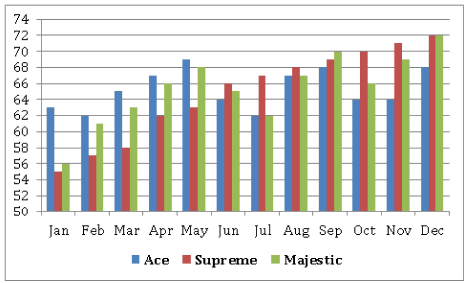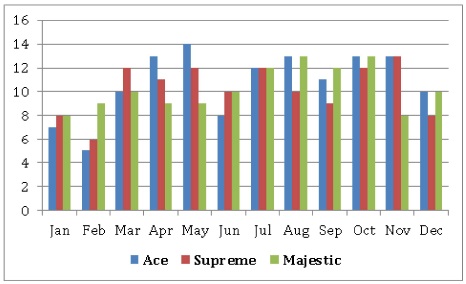The first graph above shows the monthly sales (in Rs. lakhs) and the second graph shows the percentage profit on a monthly basis.

Q.What was the maximum annual profit by any division (in Rs. lakhs) for the given year?

Solution:

In isolation, this question should not be attempted as calculating the annual profit is too tedious (36 calculations - 12 months x 3 divisions). However, observe that each question in the set involves use of the monthly profit figures. Hence, if the entire set is to be attempted, then the monthly profit for each division should be calculated as given in the table below: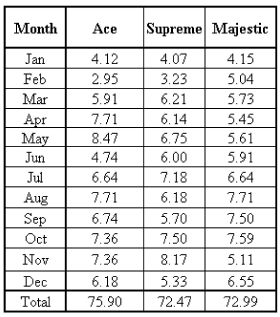Thus, the maximum annual profit is Rs. 75.9 lakhs.
Hence, option 3.

QUESTION: 35

A company - Silsila Pharmaceuticals - has three divisions. For a certain year, the CFO recorded the monthly sales and monthly percentage profit for each division as shown below:The first graph above shows the monthly sales (in Rs. lakhs) and the second graph shows the percentage profit on a monthly basis.

Q.What is the average monthly profit (in Rs. lakhs) per division for Silsila Pharmaceuticals?

Solution:

Consider the solution to the previous question.
Total annual profit = 75.9 + 72.47 + 72.99 = Rs. 221.36 lakhs

Average monthly profit (in Rs. lakhs) per division = 221.36/(12 x 3) = 6.15 Hence, option 2.

QUESTION: 36

A company - Silsila Pharmaceuticals - has three divisions. For a certain year, the CFO recorded the monthly sales and monthly percentage profit for each division as shown below:The first graph above shows the monthly sales (in Rs. lakhs) and the second graph shows the percentage profit on a monthly basis.

Q.Majestic, Supreme and Ace are located in states A, B and C respectively where the tax on profit is 8%, 11% and 10% respectively. What is the approximate profit after tax (in Rs. lakhs) for Silsila Pharmaceuticals in this year?

Solution:

Consider the solution to the earlier questions.

Total profit = Rs. 221.36 lakhs Majestic, Supreme and Ace have to pay 8%, 11% and 10% tax respectively.
Profit after tax = (0.92 x 72.99) + (0.89 x 72.47) + (0.9 x 75.9)

= 67.15 + 64.5 + 68.31
= Rs. 199.96 lakhs

Hence, option 1

QUESTION: 37

A company - Silsila Pharmaceuticals - has three divisions. For a certain year, the CFO recorded the monthly sales and monthly percentage profit for each division as shown below:The first graph above shows the monthly sales (in Rs. lakhs) and the second graph shows the percentage profit on a monthly basis.

Q.Which division has witnessed an increase in profit the maximum number of times as compared to its profit the previous month?

Solution:

Consider the solution to the first question.
The number of instances of increase in each division is:

Ace = 6; Supreme = 5 and Majestic = 8 Hence, option 2.

QUESTION: 38

in how many ways can the letters of word "INDEPENDENCE" be arranged such that no vowels are together?

Solution:

In the word INDEPENDENCE, there are 5 vowels and 7 consonants.
If we need to arrange letters of the word such that vowels are not together then, there are eight places as shown below where we can place these vowels: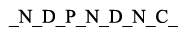Number of ways in which five vowels can be arranged in five of the eight positions: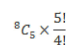Total number of ways in which the letetrs can be rearranged such that no vowels are together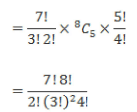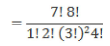Hence, option 5.

QUESTION: 39

If 31st January 2016 will be a Sunday, 1st April in that year will be on a

Solution:

2016 will be a leap year since 2016 is divisible by 4.
Number of days from 1st February 2016 to 1st April 2016 = 29+ 31 + 1 = 61 = 7(8) + 5
Thus, there are 5 odd days in the given period.
Hence, 1st April 2016 will be on the 5th day after Sunday i.e. on Friday.

Hence, option 4

QUESTION: 40

The highest power of 8 in 140! is:

Solution:

The highest power of 8 in 140! corresponds to the number of 8 s in 140!.
8 = 23
So, the number of 8s in 140! can be found by calculating the number of 2s in 140! and then grouping them in the form ( 2 x 2 x 2 ) .
The number of 2s in 140! is: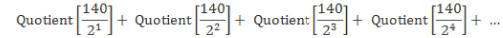70 + 35 + 17 + 8 + 4 + 2 + 1
= 137
In the above case, there are seven terms because the quotient can be found only till the divisor is 27. When the divisor is 28 i.e. 256, the quotient is 0.
Thus, there are 137 2s in 140!
Group these in the form ( 2 x 2 x 2 ) . 137 = (45 x 3) + 2

i.e. 137 2s can be written as 45 groups of 8 with 2 2s left over.
Thus, there are 45 8s in 140!
Thus, the highest power of 8 in 140! is 45.
Hence, option 4.

QUESTION: 41

What is the area of the figure bounded by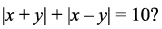Solution: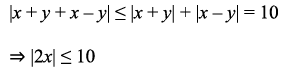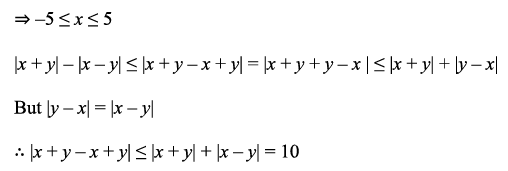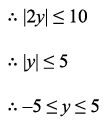Thus, we get the graph as: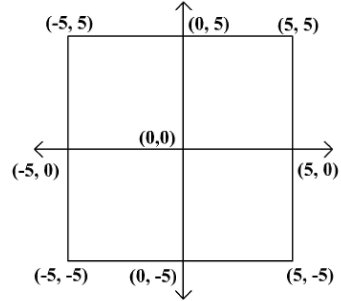Required area = Area of square of side 10 = 102 = 100

Hence, option 1.

QUESTION: 42

Group Question

Answer the following question based on the information given below.

The table below shows the number of two wheelers and four wheelers in different states of country X.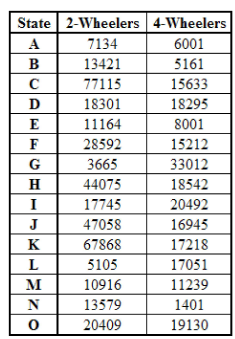Q.What is the approximate ratio of the number of two wheelers to that of four wheelers in the state which has the highest four wheelers to two wheelers ratio?

Solution:

By observation we can see that the highest ratio of four wheelers to two wheelers is in state G and is approximately equal to 9 : 1

The ratio o f two wheelers to four wheelers for that state = 1 : 9

Hence, option 4.

QUESTION: 43

The table below shows the number of two wheelers and four wheelers in different states of country X.Q.Any state is classified as a grade A-state provided that the ratio of the number of four wheelers to two wheelers, is more than 1. Based on this how many states are not graded as A?

Solution:

Except the states G, I, L and M none of the other states are graded as A.
The number of states that are not graded A = 15 - 4 = 11

Hence, option 1.

QUESTION: 44

The table below shows the number of two wheelers and four wheelers in different states of country X.Q.If the percentage share of company 1 in the total number of two wheelers in the given states is 58.50% and that of company 2 is 31.60%, then which of the following statements is/are true?

A. State K accounts for the maximum number of company 1 two wheelers in the given states.
B. The difference in the number of two wheelers of company 2 and company 1 in the given states is approximately equal to the total number of vehicles in state B and K put together.
C. The total number of vehicles in the states D, F and G put together is 11707
7.

Solution:

We cannot validate the veracity of A as the distribution of company 1 two wheelers in the states is not given.
The statements B and C cannot be verified as we do not know whether vehicles constitute only two and four wheelers or have other types too.
Hence, option 5.

QUESTION: 45

The table below shows the number of two wheelers and four wheelers in different states of country X.Q.The states are ranked based on the number of two wheelers they have and also based on the number of four wheelers they have, both in an ascending order. Which of the following statements is/are true?

Solution:

Arranging the states in the orders stated, we can see that states A and H hold the same ranks (ranks 3 and 12 respectively) in both the lists.
Hence, option 4.

QUESTION: 46

The square of the impact of a meteorite hitting the earth is directly proportional to the cube of its weight. When the weight of meteorite was 2500 tons, the impact was 1500 KiloN. If the meteorite breaks into three parts with their weights in the ratio 9 : 9 : 4 and 300 tons was lost (as energy) due to heat, then what will be loss in impact (in KiloN) due to the breaking of meteorite?

Solution:

(Impact)2 = constant x (weight)3 ...(i)

If weight = 2500 ton, Impact = 1500 KiloN

Substituting the values in (i):

(1500)2 = constant x (2500)3

Taking Square root on both the sides: Let (constant)1/2 = k

1500 = k x (2500)3/2

1500 = k x 125000

⇒ k =3/250

There was a loss of 300 tons due to breaking of meteorite, therefore net weight of 3 parts of meteorite = (2500 - 300) tons =2200 tons

Since the ratio of weights of three parts of meteorites is 9 : 9 : 4, weights of the three parts will be 900 tons, 900 tons and 400 tons respectively.

The net impact of the three broken up meteorites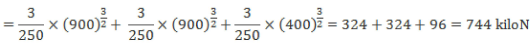Loss in impact = 1500 - 744 = 756 kiloN

Hence, option 4.

QUESTION: 47

What is the equation of a line passing through a point with polar coordinates (4, 60°) and parallel to perpendicular bisector of a line passing through origin and a point with polar coordinates (6, 60°)?

Solution: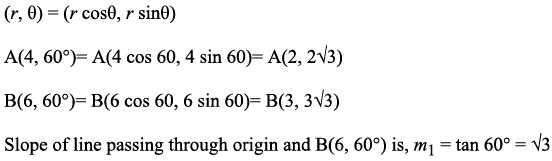Let the slope of the perpendicular bisector of line passing through B(6, 60°) and origin be m2.

m1 x m2 = - 1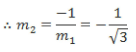Since the perpendicular bisector is parallel to line passing through points, slope of line passing through point A is equal to the slope of perpendicular bisector.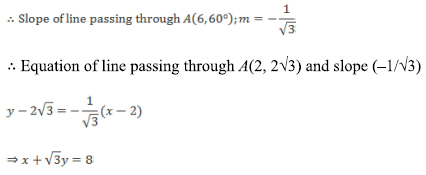Hence, option 3.

QUESTION: 48

If the value of a + c = 1/2, b = 1 and ac = - 2 , what is the value of (a2 - b2 + c2)?

Solution:

a + c = 1/2, b= 1 and ac = -2

Now, (a + c + b) (a + c - b) = a2 - b2 + c2 + 2ac

a2 - b2 + c2 = (a + c)2 - b2 - 2ac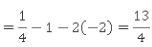Hence, option 2.

QUESTION: 49

What will be the next number in the series?

257 , 327 , 369 , 531 , 546 , ____

Solution:

The difference between successive terms is 70, 42, 162 and 15.

Thus, there is no pattern to the difference.
However, notice that 70 = 2 x 5 x 7 Thus, 327 = 257 + (2 x 5 x 7)

Similarly, 369 = 327 + 42 = 327 + (3 x 2 x 7)

Thus, the pattern is: difference between consecutive terms = product of digits of the term
Hence, missing term = 546 + (5 x 4 x 6) = 546 + 120 = 666

Hence, option 3.

QUESTION: 50

Given below are two statements. Analyze them and mark the option that correctly states their relationship.
A. 21st December is the shortest day of the year in the northern hemisphere.
B. The inclination of the earth and its distance from the sun is unique on 21st December.

Solution:

These two statements are definitely related to a common topic - the peculiarity of 21st December. Since the inclination of the earth and its distance from the sun on 21st December is unique, this day appears to be the shortest day of the year in the northern hemisphere. This means that statement B is the cause of statement A.
Hence, the correct answer is option 2.

QUESTION: 51

Read the following situation and choose the best possible alternative.

Mr. Arora, the marketing head of a new FMCG based company with limited reach, has to take a call between direct and indirect marketing of the company’s latest product ‘Serenade Hand Wash’. Whereas direct marketing involves the much tried and tested path of advertising and consumer awareness, indirect marketing deals with striking deals with low level distributors to increase inventory stocks of the company’s product so it is sold more as compared to other companies’ products. Obvious to say, indirect marketing is risky but cheaper than direct marketing.

Q.What approach should Mr. Arora implement and why?

Solution:

The first sentence of the case study is the one that clinches the entire argument. The FMCG industry works on branding and for any new company to make its mark, it has to spend a lot of money on increasing brand consciousness/awareness among its consumers.
Option 2 is the correct option as it takes care of this aspect of marketing. Option 1 is incorrect because of its simplistic nature. The advantage of direct marketing has been mentioned but it has not been effectively offset by the advantage of indirect marketing.
Option 3 assumes that Mr. Arora’s company does not have an advertising budget. A new FMCG company has no choice but to advertise since its products are consumer products.
Option 4 falls for the same errors of misconception. There is nothing that proves that indirect marketing is unique and innovative.
Option 5 also relies on a misconception; just because something looks good in theory does not mean there are no risks in its real-world implementation. Hence, the correct answer is option 2.

QUESTION: 52

Consider the pattern given below: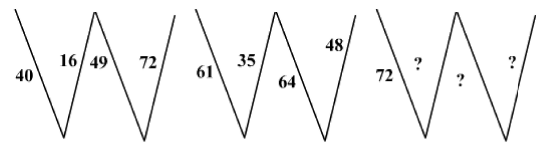Q.Which of the following will complete the third pattern?

Solution:

The given patterns used in each figure:

a2 - b2; ( a + b )2;2ab

For the 1st diagram: 42 - 02 = 16; (1 + 6 )2 = 49; 2 x 4 x 9 = 72

For the 2nd diagram: 62 - 12 = 35; (3 + 5 )2 = 64; 2 x 6 x 4 = 48
So,
For the 3rd diagram: 72 - 22 = 45 : ( 4 + 5)2 = 81 ; 2 x 8 x 1 = 16

Hence, the correct answer is option 2

QUESTION: 53

Neo, a robot on the moon searching for water, faces north and walks for 10 meters. He then turns right and walks for 6 meter. Again he turns right and walks for 8 meters, after which he turns left and walks for 4 meters. He then turns left again and walks for 5 meters, after which he turns right and walk for 2 meters, then he turns left and walks for 3 meters, then he takes another left and walks for 4 meters and then finally he again turns left and walks for 4 meters and finds water.

Q.What is the minimum distance (in meters) between Neo's source and destination?

Solution: